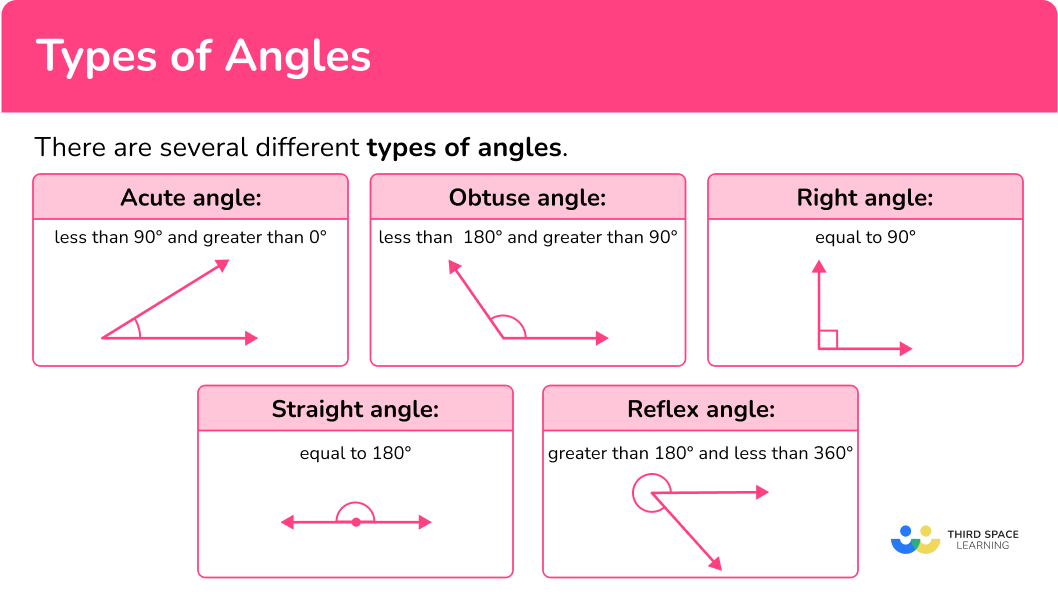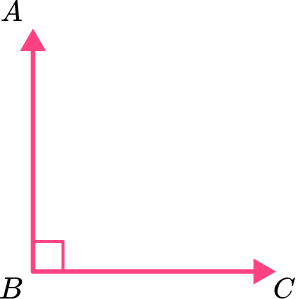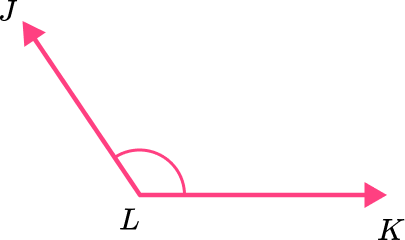Math resources Geometry Angles

Types of angles

# Types of angles

Here is everything you need to know about the different types of angles. You will learn how to identify angles and classify them.

Students first learn about angles in 4th grade with their work in geometric measurements. They expand that knowledge as they progress through middle school.

## What are the different types of angles?

There are several different types of angles.

Acute angles – An acute angle is an angle that is less than 90^{\circ} and greater than 0^{\circ} .

Obtuse angles – An obtuse angle is an angle greater than 90^{\circ} but less than 180^{\circ} .

Reflex angles – A reflex angle is greater than 180^{\circ} but less than 360^{\circ}.

Right angles – A right angle is 90^{\circ} . The two arms of a right angle are perpendicular. This means that the two rays or lines that meet at the vertex point form a right angle.

There will always be a small square by the vertex of the angle which is the notation for a right angle.

Straight angles – A straight angle is 180^{\circ} and it forms a straight line. The arms of a straight angle are rays going in opposite directions, also known as opposite rays. A straight angle is considered one-half a whole turn because it is 180^{\circ} .

Complete turn – Also known as a full rotation is 360^{\circ}. It is sometimes referred to as a full angle.

An angle is formed between two lines (rays) meeting around a common point. The rays are called the arms of the angle and the common point is called the vertex of an angle.

An angle is named by using the symbol (∠) followed by three capital letters, with the middle letter as the vertex of the angle.

Parts of an angle:

The name of the angle on the left is ∠ABC or ∠CBA , where point B is the vertex of angle.

∠ABC is 30^{\circ}

The unit of measure for an angle is the degree, which is shown with the degree sign, for example, ∠ABC is 30^{\circ} reads as “Angle ABC is thirty degrees.”

Did you know? – The word angle comes from the Latin word angulus, which means a corner.

### What are the different types of angles?## Common Core State Standards

How does this relate to 4th grade math?

• Grade 4 – Measurement and Data (4.MD.C.5.a)
An angle is measured with reference to a circle with its center at the common endpoint of the rays, by considering the fraction of the circular arc between the points where the two rays intersect the circle. An angle that turns through 1/360 of a circle is called a “one-degree angle,” and can be used to measure angles.

• Grade 4 – Measurement and Data (4.MD.C.5.b)
An angle that turns through n one-degree angles is said to have an angle measure of n degrees.

• Grade 4 – Measurement and Data (4.MD.C.7)
Recognize angle measure as additive. When an angle is decomposed into non-overlapping parts, the angle measure of the whole is the sum of the angle measures of the parts. Solve addition and subtraction problems to find unknown angles on a diagram in real world and mathematical problems, for example, by using an equation with a symbol for the unknown angle measure.

• Grade 4 – Geometry (4.G.A.1)
Draw points, lines, line segments, rays, angles (right, acute, obtuse), and perpendicular and parallel lines. Identify these in two-dimensional figures.

## How to identify the type of angle

In order to find the angle type:

1. Recall the definitions of the types of angles.
2. Explain how the angle fits the definition.

## Types of angles examples

### Example 1: classify angle given a diagram

What type of angle is ∠ABC?

1. Recall the definitions of the types of angles.

2Explain how the angle fits the definition.

Since ∠ABC is less than 90^{\circ} it must be an acute angle.

An acute angle is an angle whose measure is greater than 0^{\circ} and less than 90^{\circ} .

### Example 2: classify angle given a diagram

What type of angle is ∠KMN?

Recall the definitions of the types of angles.

Explain how the angle fits the definition.

### Example 3: classify angle given a diagram

What type of angle is ∠QRS?

Recall the definitions of the types of angles.

Explain how the angle fits the definition.

### Example 4: classify angle given a measure

Classify the angle:

∠DEF is 122^{\circ} .

Recall the definitions of the types of angles.

Explain how the angle fits the definition.

### Example 5: classify angle given a measure

Classify the angle:

∠PQR is 208^{\circ} .

Recall the definitions of the types of angles.

Explain how the angle fits the definition.

### Example 6: classify angles

Classify the angle:

∠JKL is 180^{\circ} .

Recall the definitions of the types of angles.

Explain how the angle fits the definition.

### Teaching tips for types of angles

• Have students draw and create the different types of angles using colored pencils.

• Use digital platforms for students to practice with different types of angles.

• Have students create designs using the different types of angles.

• Instead of giving students practice worksheets, have them either sketch the different types of angles or use digital platforms for game playing.

### Easy mistakes to make

• Confusing an acute angle and an obtuse angle
For example thinking that an angle that measures 74^{\circ} is obtuse instead of acute.

• Naming the angle incorrectly
For example, naming the angle as ∠CBA instead of ∠CAB. Point A is the vertex, so it should be the middle letter.

• Forgetting the degree sign
When measuring or estimating angles, your answer must always be given in degrees.

### Types of angles practice questions

1. Classify the angle in the diagram below:Acute angleReflex angleRight angleObtuse angleThe vertex of ∠ABC is where the rays meet at the square symbol, which means that the angle is 90^{\circ} and a right angle.

2. Classify the angle in the diagram below:Obtuse angleAcute angleRight angleReflex angleJLK is greater than 90^{\circ}, and less than 180^{\circ}, so it is an obtuse angle.

3. Classify the angle in the diagram.Straight angleRight angleObtuse angleAcute angleThe angle is equal to 90^{\circ} , so it is a right angle.

4. ∠LMN measures 34^{\circ}, what type of angle is it?

Right angleReflex angleObtuse angleAcute angle∠LMN is 34^{\circ} , which is greater than 0^{\circ} and less than 90^{\circ} , so it is an acute angle.

5. ∠QRS is 180^{\circ}, what type of angle is it?

Right angleStraight angleAcute angleObtuse angle∠QRS is 180^{\circ} is equal to 180^{\circ} , so it is a straight angle.

6. ∠RST is 170^{\circ}, what type of angle is it?

Straight angleStraight angleObtuse angleAcute angle∠RST is 170^{\circ} , which is greater than 90^{\circ} and less than 180^{\circ} , so it is an obtuse angle.

## Types of angles FAQs

Are all angles measured in degrees?

No, angles can be measured in degrees or in radians. However, radian measures are what you will use in high school.

Are angle measurements only positive?

No, angles can have positive or negative measurements. However, in elementary and middle school, you will only work with positive angles, and negative angle measurements are what you will encounter in high school.

How do you measure angles?

Protractors are tools used for measuring angles.

Why is it necessary to learn types of angles?

Learning about types of angles is necessary to be able to classify polygons, such as triangles and quadrilaterals.

What are vertical angles?

Vertical angles are formed by intersecting lines.

What are supplementary angles and complementary angles?

Supplementary angles are a pair of angles whose sum is 180^{\circ} and complementary angles are a pair of angles whose sum is 90^{\circ}.

## Still stuck?

At Third Space Learning, we specialize in helping teachers and school leaders to provide personalized math support for more of their students through high-quality, online one-on-one math tutoring delivered by subject experts.

Each week, our tutors support thousands of students who are at risk of not meeting their grade-level expectations, and help accelerate their progress and boost their confidence.